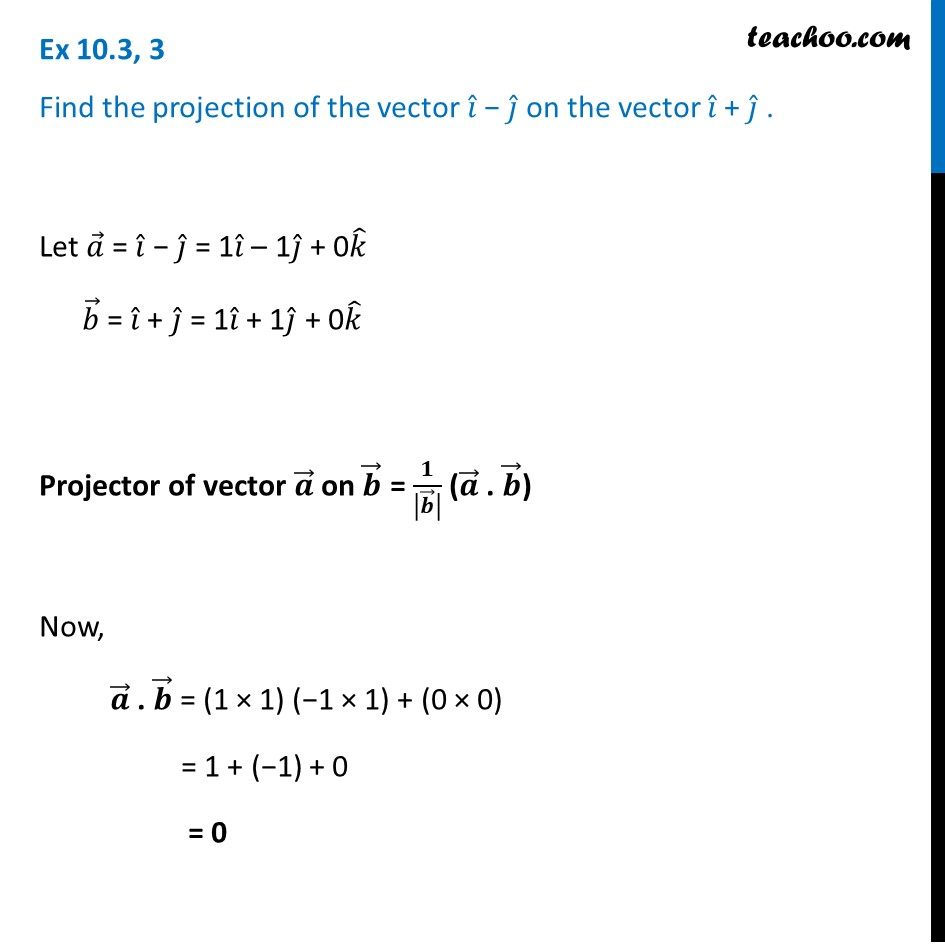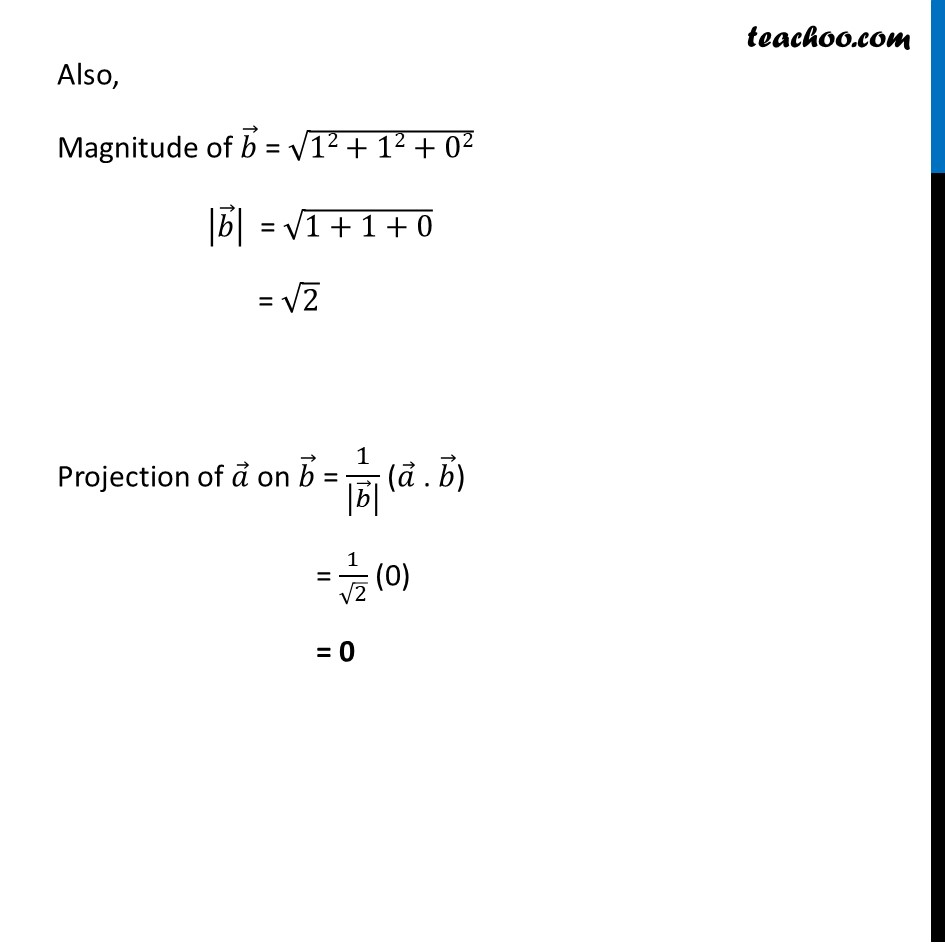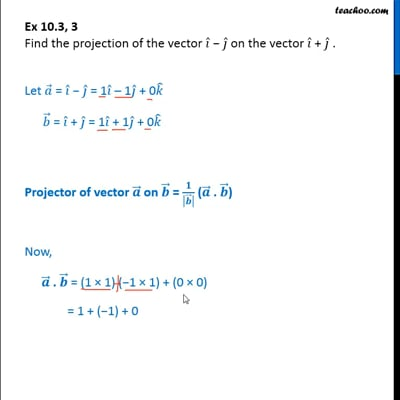Scalar product - Projection

Chapter 10 Class 12 Vector Algebra
Concept wiseThis video is only available for Teachoo black users

Introducing your new favourite teacher - Teachoo Black, at only ₹83 per month

### Transcript

Ex 10.3, 3 Find the projection of the vector 𝑖 ̂ − 𝑗 ̂ on the vector 𝑖 ̂ + 𝑗 ̂ .Let 𝑎 ⃗ = 𝑖 ̂ − 𝑗 ̂ = 1𝑖 ̂ – 1𝑗 ̂ + 0𝑘 ̂ 𝑏 ⃗ = 𝑖 ̂ + 𝑗 ̂ = 1𝑖 ̂ + 1𝑗 ̂ + 0𝑘 ̂ Projector of vector 𝒂 ⃗ on 𝒃 ⃗ = 𝟏/|𝒃 ⃗ | (𝒂 ⃗ . 𝒃 ⃗) Now, 𝒂 ⃗ . 𝒃 ⃗ = (1 × 1) (−1 × 1) + (0 × 0) = 1 + (−1) + 0 = 0 Also, Magnitude of 𝑏 ⃗ = √(12+12+02) |𝑏 ⃗ | = √(1+1+0) = √2 Projection of 𝑎 ⃗ on 𝑏 ⃗ = 1/|𝑏 ⃗ | (𝑎 ⃗ . 𝑏 ⃗) = 1/√2 (0) = 0